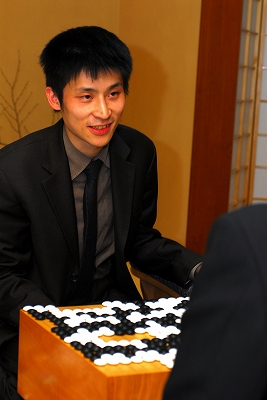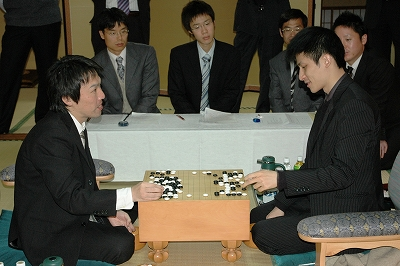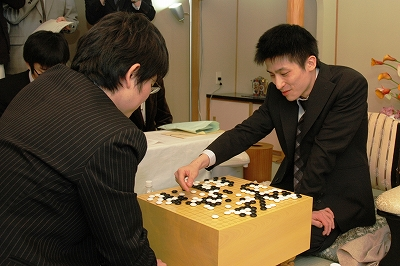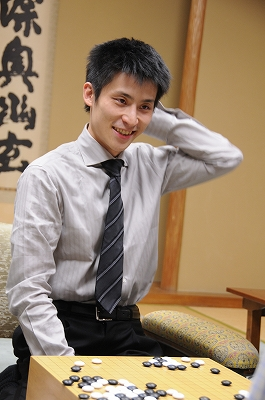## Archive for November, 2009

### Kim Jiseok Eliminated From Nongshim Cup

Sunday, November 29th, 2009

After eliminating three opponents in a row, Kim Jiseok of South Korea finally had to surround to Xie He of China.The game record is here. Xie He (white) won by resignation.

(; RE[W+R] BR[6d] EV[11th Nongshim Cup 4th round] WR[7d] PB[Kim Jiseok] FF SZ GM KM[6.5] DT[2009-11-28] PW[Xie He] ;B[qd];W[dd];B[pq];W[dp];B[fc];W[cf];B[db];W[oc];B[cc];W[po];B[qo] ;W[qn];B[qp];W[pn];B[nq];W[qg];B[pe];W[qj];B[ch];W[ff];B[ck];W[cm] ;B[gd];W[dj];B[eh];W[cj];B[ef];W[dg];B[dh];W[eg];B[fg];W[gg];B[fh] ;W[fe];B[dk];W[bj];B[dm];W[bl];B[fk];W[dn];B[ig];W[hf];B[gh];W[if] ;B[hg];W[gf];B[pj];W[pp];B[qq];W[qk];B[jf];W[je];B[ie];W[ec];B[eb] ;W[fd];B[id];W[cd];B[jg];W[jd];B[ic];W[qb];B[mc];W[md];B[lc];W[nd] ;B[of];W[ld];B[jc];W[mg];B[ph];W[qh];B[nj];W[nh];B[oh];W[mj];B[mk] ;W[ni];B[pk];W[nk];B[ql];W[rl];B[nl];W[oj];B[qi];W[ri];B[pi];W[rk] ;B[lk];W[kj];B[le];W[nf];B[kd];W[ml];B[nm];W[kl];B[ok];W[nj];B[no] ;W[ll];B[dq];W[cq];B[eq];W[cr];B[en];W[do];B[be];W[bd];B[bf];W[bg] ;B[cg];W[df];B[ag];W[bc];B[hq];W[jq];B[io];W[jo];B[jn];W[ko];B[in] ;W[kn];B[jk];W[kk];B[ob];W[pb];B[nb];W[pd];B[qf];W[pg];B[og];W[np] ;B[rg];W[rh];B[re];W[rc];B[rd];W[pc];B[pf];W[op];B[mq];W[sg];B[sf] ;W[rf];B[mp];W[mo];B[rg];W[oq];B[or];W[rf];B[bb];W[se];B[dc];W[fp] ;B[fq];W[em];B[fm];W[el];B[ek];W[fn];B[fl];W[gp];B[lo];W[mn];B[ir] ;W[jr];B[hp];W[ro];B[rp];W[pr];B[nr];W[sn];B[bk];W[ak];B[sp];W[lp] ;B[gn];W[eo];B[jj];W[ji];B[ii];W[jl];B[il];W[im];B[ik];W[jm];B[hm] ;W[dr];B[lr];W[jh];B[lg];W[lh];B[me];W[ne];B[mf];W[nc])

Complete tournament table is here.

### 11th Nongshim Cup: Kim Jiseok Eliminated Takao Shinji

Friday, November 27th, 2009

Kim Jiseok of South Korea eliminated three opponents in a row in the 11th Nongshim Cup after defeating Takao Shinji 9p of Japan. He will be matched next with one of the remaining Chinese players the day after tomorrow, in the last day of the first stage of Nongshim Cup.Kim Jiseok (white) won by half a point.

(;CA[Windows-1252]SZAP[MultiGo:4.4.4]EV[11th Nongshim Cup]DT[2009-11-27]PB[Takao Shinji] BR[9p]BT[Japan]PW[Kim Jiseok]WR[6p]WT[South Korea]KM[6.5]HARE[W+0.5]MULTIGOGM ;B[pd];W[dd];B[qp];W[dq];B[oq];W[qf];B[nc];W[rd];B[qg];W[pg];B[qh];W[pf];B[qc];W[ph] ;B[qj];W[qi];B[ri];W[pi];B[do];W[co];B[cn];W[cp];B[fc];W[cf];B[db];W[dn];B[cm];W[en] ;B[cj];W[cc];B[jq];W[hc];B[ed];W[dc];B[cb];W[de];B[bc];W[bd];B[bb];W[ec];B[eb];W[fd] ;B[gc];W[gd];B[hd];W[hb];B[gb];W[jc];B[pj];W[rh];B[rg];W[sh];B[rk];W[rf];B[lc];W[je] ;B[gq];W[oo];B[np];W[pp];B[pq];W[qo];B[rp];W[mn];B[pn];W[on];B[qn];W[nj];B[he];W[ee] ;B[ig];W[ej];B[ch];W[eh];B[fg];W[md];B[ld];W[le];B[me];W[lf];B[ge];W[ga];B[fb];W[fe] ;B[ii];W[gi];B[ik];W[bi];B[bj];W[bh];B[bg];W[cg];B[ci];W[bf];B[bo];W[bp];B[ao];W[jp] ;B[iq];W[lp];B[in];W[kn];B[ne];W[am];B[bm];W[ho];B[kq];W[jj];B[ij];W[oe];B[nd];W[hh] ;B[ih];W[ap];B[al];W[bl];B[cl];W[ak];B[aj];W[dk];B[bk];W[bn];B[al];W[an];B[el];W[hg] ;B[if];W[gk];B[gj];W[fk];B[ck];W[hn];B[gh];W[fi];B[hi];W[kd];B[eg];W[lq];B[kp];W[ko] ;B[er];W[lb];B[kb];W[kc];B[mc];W[kh];B[ki];W[ji];B[im];W[hl];B[kg];W[kj];B[il];W[io] ;B[lg];W[li];B[km];W[kl];B[mg];W[mb];B[nb];W[jb];B[lm];W[ol];B[nh];W[ql];B[rl];W[jf] ;B[jg];W[nf];B[oj];W[rn];B[rm];W[oi];B[nk];W[ml];B[mk];W[ll];B[jn];W[jo];B[mj];W[ni] ;B[mi];W[qm];B[ro];W[kr];B[jr];W[mr];B[dr];W[cr];B[fq];W[ds];B[fo];W[fn];B[eq];W[eo] ;B[ep];W[go];B[ks];W[lr];B[hr];W[mm];B[si];W[sg];B[nr];W[rc];B[rb];W[qd];B[qb];W[lh] ;B[mh];W[pm];B[sn];W[po];B[dp];W[cq];B[bo];W[ao];B[es];W[cs];B[od];W[dl];B[dm];W[em] ;B[pe];W[hk];B[id];W[mf];B[of];W[og];B[kf];W[ke];B[ok];W[ns];B[os];W[sb];B[hj];W[rn] ;B[no];W[ka];B[ma];W[ls];B[js];W[ps];B[ms];W[dg];B[gf];W[ns];B[or];W[qn];B[fa];W[ha] ;B[ic];W[ib];B[na];W[la];B[dj];W[ek];B[hm];W[gm];B[qe];W[re];B[ad];W[ae];B[ac];W[ag] ;B[ai];W[ah];B[fp];W[hp];B[hq];W[fh];B[gg];W[nl];B[nn];W[nm];B[mo];W[lo];B[ra];W[ef] ;B[ie];W[dh];B[di];W[ms];B[oe];W[op];B[nq])

Complete tournament table is here.

### 11th Nongshim Cup: Kim Jiseok Eliminated Ding Wei

Thursday, November 26th, 2009

In the 11th Nongshim Cup Kim Jiseok of South Korea eliminated Ding Wei 9p of China. He will play next against Takao Shinji of Japan.The game record is here. Kim Jiseok (white) won by resignation.

(; RE[W+R] BR[9d] EV[11th Nongshim Cup 2nd round] WR[6d] PB[Ding Wei] FF SZ GM KM[6.5] DT[2009-11-26] PW[Kim Jiseok] ;B[qd];W[dc];B[pp];W[dp];B[de];W[ce];B[cf];W[cd];B[od];W[qn];B[pk] ;W[nq];B[pn];W[pm];B[on];W[qp];B[qo];W[ro];B[po];W[rm];B[qq];W[rp] ;B[om];W[jp];B[cn];W[fq];B[pl];W[pq];B[qm];W[oq];B[rn];W[rq];B[jd] ;W[df];B[cg];W[ef];B[dj];W[le];B[lc];W[qc];B[rd];W[mc];B[ld];W[ne] ;B[ke];W[md];B[lf];W[oc];B[pc];W[pb];B[pd];W[lb];B[nb];W[ob];B[nd] ;W[nc];B[me];W[kb];B[qb];W[qa];B[jn];W[ij];B[eh];W[kj];B[mi];W[mj] ;B[li];W[lj];B[nj];W[ln];B[ko];W[mo];B[jk];W[jl];B[km];W[dk];B[ck] ;W[cl];B[dl];W[ek];B[bl];W[ml];B[kl];W[ik];B[il];W[nk];B[hl];W[oj] ;B[ni];W[ok];B[ph];W[ki];B[kp];W[co];B[gq];W[gr];B[hq];W[fp];B[hr] ;W[pi];B[qi];W[oh];B[mg];W[qh];B[pg];W[qj];B[ri];W[qg];B[oi];W[pj] ;B[rk];W[rj];B[sj];W[sn];B[rl];W[pf];B[og];W[qn];B[rb];W[qk];B[ql] ;W[qf];B[si];W[rh];B[rn];W[of];B[ng];W[qn];B[mm];W[lm];B[sh];W[sg] ;B[sk];W[ic];B[mn];W[ll];B[lo];W[no];B[rn];W[gj];B[ej];W[qn];B[ol] ;W[nn];B[nm];W[rf];B[rn];W[se];B[qr];W[rr];B[re];W[qn];B[sd];W[rn] ;B[sf];W[fk];B[kk];W[hh];B[rg];W[he];B[mp];W[lr];B[gg];W[dn];B[bn] ;W[gf];B[cq];W[bo];B[bp];W[an];B[dm];W[en];B[br];W[dr];B[dq];W[er] ;B[ap];W[ao];B[cr];W[cj];B[bk];W[op];B[hg];W[ih];B[hj];W[fg];B[gh] ;W[hi];B[eq];W[ep];B[gn];W[em];B[hf];W[ge];B[hk];W[gi];B[fh];W[jj] ;B[kr];W[lq];B[kq];W[sg];B[jc];W[jb];B[rg];W[cp];B[aq];W[sg];B[id] ;W[hd];B[rg];W[ci];B[fj];W[gk];B[am];W[dg];B[ch];W[dh];B[di];W[fi] ;B[ei];W[bf];B[bh];W[sg];B[hc];W[ib];B[ls];W[se];B[ns];W[mr];B[ms] ;W[pr];B[sf];W[el];B[cm];W[se];B[mb];W[oo];B[sf];W[rg])

Complete tournament table is here.

### Cho U Keeps Oza Title

Thursday, November 26th, 2009

Cho U defeated challenger Yamada Kimio with 3 straight wins and kept his Oza title for another year.The 3rd game record is here. Cho U (white) won by 3.5 points.

(; KM[6.5] EV[57th Oza title match 3] FF SZ GM PW[Cho U] WR[Oza] DT[2009-11-26] BR[9d] RE[W+3.5] PB[Yamada Kimio] ;B[pd];W[dd];B[pq];W[dq];B[do];W[cm];B[cq];W[gq];B[dp];W[dr];B[bo] ;W[cj];B[ip];W[cr];B[gp];W[fp];B[fo];W[ep];B[eo];W[hp];B[go];W[hq] ;B[ho];W[iq];B[jp];W[jq];B[kp];W[lq];B[ch];W[ej];B[eh];W[gc];B[gi] ;W[cf];B[ic];W[gk];B[ie];W[qf];B[qh];W[kc];B[ge];W[nc];B[pc];W[ph] ;B[pi];W[pg];B[qj];W[ne];B[re];W[qe];B[rd];W[nh];B[dl];W[el];B[bd] ;W[cc];B[ce];W[de];B[bf];W[dm];B[po];W[qm];B[qo];W[ri];B[qi];W[nj] ;B[pl];W[nl];B[pm];W[fh];B[fi];W[ei];B[eg];W[ff];B[fg];W[gf];B[ke] ;W[cd];B[be];W[ef];B[bi];W[ig];B[md];W[nd];B[lb];W[ob];B[rf];W[jb] ;B[lc];W[ib];B[kd];W[lf];B[jg];W[le];B[ld];W[jf];B[hg];W[jh];B[hf] ;W[gg];B[kg];W[if];B[hh];W[kf];B[gh];W[hc];B[id];W[bq];B[hl];W[gl] ;B[hm];W[jj];B[mr];W[lr];B[kl];W[cg];B[bg];W[bj];B[bc];W[bb];B[ab] ;W[cb];B[fd];W[fc];B[lk];W[hk];B[ki];W[ji];B[ai];W[il];B[im];W[jl] ;B[jm];W[or];B[mi];W[ni];B[mq];W[lp];B[np];W[ko];B[ln];W[lo];B[mn] ;W[no];B[mo];W[mp];B[nq];W[kn];B[km];W[nn];B[ml];W[ol];B[kh];W[pk] ;B[qk];W[on];B[mm];W[pn];B[ok];W[nk];B[qn];W[jn];B[mg];W[mh];B[lg] ;W[ng];B[mf];W[me];B[in];W[oj];B[ql];W[ms];B[ns];W[ls];B[ih];W[pb] ;B[qb];W[rb];B[qc];W[qa];B[rc];W[qg];B[rg];W[kj];B[lj];W[hj];B[nr] ;W[op];B[oq];W[oo];B[pp];W[cp];B[co];W[fm];B[gm];W[fn];B[bm];W[bl] ;B[am];W[bp];B[ao];W[pe];B[ed];W[ec];B[kq];W[kr];B[io];W[kb];B[ka] ;W[je];B[jd];W[ac];B[ad];W[ci];B[dh];W[df];B[na];W[nb];B[bh];W[kk] ;B[oi];W[nf];B[li];W[pj];B[di];W[dj];B[fj];W[fk];B[al];W[ak];B[aj] ;W[bk];B[cn];W[ja];B[la];W[ra];B[sb];W[oh];B[en];W[em];B[ap];W[aq] ;B[od];W[oe];B[mk];W[ba];B[gn])

Complete tournament table is here.

### 11th Nongshim Cup: Kim Jiseok Eliminated Yamashita Keigo

Wednesday, November 25th, 2009

The 11th Nongshim Cup started with Kim Jiseok 6p of S. Korea eliminating Yamashita Keigo 9p (and Kisei title holder) of Japan.Here are the players from each of the three participating countries:

Korea: Lee Changho(9p), Park Yeonghun(9p), Yun Junsang(7p), Kim Jiseok(6p), Kim Seongjae(3p)

China: Chang Hao(9p), Ding Wei(9p), Gu Li(9p), Xie He(7p), Liu Xing(7p)

Japan: Yamada Kimio(9p), Hane Naoki(9p), Takao Shinji(9p), Yamashita Keigo(9p), Iyama Yuta(9p)

Next game will be played tomorrow (or tonight in the US) with Kim Jiseok being matched against one of the Chinese players.

Tournament table here.

### Cho U – Yamada Kimio 2:0 in Oza Title

Wednesday, November 25th, 2009

Cho U, Oza title holder, won the second game as well against challenger Yamada Kimio. Cho U is just one win away from keeping this title for another year.The game record is here. Cho U (black) won by resignation.

(; DT[2009-11-24] PB[Cho U] RE[B+R] PW[Yamada Kimio] FF SZ GM BR[Oza] KM[6.5] EV[57th Oza title match 2] WR[9d] ;B[pd];W[dd];B[qp];W[dp];B[fq];W[hq];B[nq];W[qj];B[cn];W[fp];B[cq] ;W[dq];B[cp];W[do];B[cr];W[dr];B[fc];W[cf];B[db];W[qf];B[nd];W[ic] ;B[cc];W[lc];B[ld];W[md];B[me];W[mc];B[ne];W[kd];B[qe];W[qm];B[nc] ;W[rf];B[le];W[cl];B[dn];W[ci];B[jd];W[jc];B[ke];W[id];B[kc];W[kb] ;B[pm];W[ql];B[iq];W[ip];B[jp];W[hp];B[jr];W[jo];B[hr];W[kp];B[jq] ;W[mq];B[ko];W[np];B[oq];W[lp];B[gq];W[gp];B[eo];W[ep];B[jn];W[io] ;B[kn];W[lr];B[fs];W[cs];B[mo];W[mp];B[on];W[co];B[nr];W[po];B[oo] ;W[bo];B[qn];W[rn];B[qo];W[el];B[hm];W[rd];B[fm];W[em];B[be];W[ce] ;B[di];W[dh];B[ei];W[cj];B[oj];W[ph];B[pk];W[qk];B[pg];W[gk];B[ik] ;W[qh];B[bf];W[bg];B[oh];W[ii];B[hj];W[hh];B[cd];W[jj];B[jk];W[lj] ;B[kk];W[pl];B[ol];W[nk];B[ok];W[ni];B[oi];W[af];B[ie];W[gd];B[de] ;W[df];B[ee];W[gc];B[ef];W[eh];B[fh];W[gg];B[eg];W[ch];B[hi];W[ih] ;B[kj];W[ki];B[ge];W[fe];B[ff];W[he];B[fd];W[hf];B[rm];W[ro];B[rp] ;W[rl];B[qc];W[lh];B[dg];W[cg];B[lk];W[mk];B[mj];W[nj];B[li];W[mi] ;B[lj];W[mg];B[ml];W[rc];B[qb];W[nb];B[re];W[se];B[ob];W[fb];B[so] ;W[sm];B[bd];W[fg];B[fj];W[gf];B[dc];W[fe];B[ed];W[eb];B[ec];W[fn] ;B[dk];W[dj];B[ek])

The next game will be played on November 26th. Complete tournament table is here.

### Cho U Evens the Score in Tengen Title Match

Thursday, November 19th, 2009

Cho U, Tengen title holder, defeated the challenger Yamashita Keigo in the second game.The game record is here. Cho U, playing black, won by resignation.

(; KM[6.5] EV[35th Tengen title match 1] FF SZ GM PW[Yamashita Keigo] WR[Kisei] DT[2009-11-19] BR[Tengen] RE[B+R] PB[Cho U] ;B[pd];W[dp];B[qp];W[dc];B[fq];W[cn];B[lq];W[de];B[pj];W[nc];B[lc] ;W[qc];B[qd];W[pc];B[od];W[nd];B[oc];W[ob];B[pb];W[qb];B[nb];W[pa] ;B[ne];W[mb];B[me];W[ip];B[fo];W[in];B[dr];W[cq];B[fm];W[il];B[op] ;W[gp];B[ep];W[en];B[fn];W[gq];B[do];W[co];B[dq];W[cp];B[jo];W[jn] ;B[ko];W[kq];B[kr];W[jq];B[lp];W[fr];B[em];W[dn];B[eo];W[fk];B[dl] ;W[bl];B[ck];W[bk];B[gk];W[gl];B[io];W[hn];B[ho];W[go];B[gn];W[jr] ;B[er];W[gr];B[di];W[fi];B[bj];W[cg];B[lr];W[ir];B[dg];W[ch];B[dh] ;W[df];B[ci];W[fg];B[ek];W[fl];B[ej];W[fj];B[br];W[cr];B[cs];W[bq] ;B[bf];W[cf];B[bh];W[rp];B[ro];W[qo];B[po];W[qn];B[rq];W[oh];B[qh] ;W[of];B[qf];W[jd];B[mc];W[na];B[nj];W[mh];B[md];W[ql];B[qk];W[rk] ;B[rj];W[ok];B[oj];W[om];B[nl];W[pk];B[qj];W[pn];B[lj];W[oo];B[pp] ;W[mk];B[nk];W[rn];B[sp];W[sl];B[ol];W[pl];B[no];W[on];B[sn];W[rm] ;B[sj];W[sk];B[je];W[ie];B[jf];W[li];B[id];W[jc];B[jh];W[ig];B[if] ;W[he];B[hf];W[ge];B[kj];W[bg];B[ag];W[mj];B[ll];W[ki];B[ji];W[jj] ;B[kl];W[jg];B[kg];W[kf];B[hg];W[ih];B[kh];W[lf];B[hi];W[ke];B[kd] )

Next game will be played on December 3rd.

### Cho U Kisei Challenger

Monday, November 16th, 2009

Cho U defeated O Rissei in the playoff to decide the Kisei challenger. The Kisei title holder is Yamashita Keigo, who is currently challenging Cho U in Tengen title.The game record is here.

(; GM KM[6.5] SZ FF WR[Judan] EV[34th Kisei challenger decision match] DT[2009-11-16] PB[O Rissei] RE[W+R] PW[Cho U] BR[9d] ;B[pd];W[dd];B[qp];W[op];B[dp];W[qq];B[kq];W[rp];B[qo];W[ro];B[pq] ;W[pp];B[qn];W[qr];B[pj];W[nc];B[qf];W[pb];B[cf];W[fc];B[di];W[fq] ;B[eq];W[fp];B[dn];W[ip];B[ko];W[jq];B[mq];W[qm];B[on];W[pm];B[hq] ;W[hp];B[fr];W[gr];B[er];W[qc];B[gm];W[pn];B[me];W[im];B[mc];W[md] ;B[ld];W[nd];B[ne];W[lc];B[kd];W[co];B[do];W[cn];B[cp];W[ck];B[cj] ;W[bj];B[bi];W[dj];B[ci];W[bl];B[mb];W[nb];B[aj];W[bp];B[bq];W[dm] ;B[em];W[dl];B[ej];W[el];B[fl];W[ao];B[bk];W[aq];B[ar];W[br];B[ap] ;W[en];B[bo];W[fm];B[fn];W[em];B[gl];W[eo];B[km];W[jl];B[cc];W[cd] ;B[dc];W[ec];B[bd];W[be];B[bc];W[ce];B[bf];W[ic];B[gd];W[fe];B[ge] ;W[ae];B[db];W[eb];B[ba];W[jb];B[id];W[kc];B[kj];W[fj];B[fk];W[ek] ;B[ei];W[jj];B[ji];W[gj];B[hk];W[ij];B[hj];W[ii];B[hi];W[ki];B[hc] ;W[hb];B[jd];W[jc];B[jh];W[kk];B[lj];W[ih];B[lk];W[jg];B[kh];W[kg] ;B[lh];W[hg];B[if];W[ig];B[lg];W[bn];B[an];W[rj];B[rk];W[qk];B[qj] ;W[gh];B[gi];W[fg];B[fh];W[eh];B[fi];W[ri];B[ql];W[pk];B[pl];W[ok] ;B[ol];W[rl];B[rm];W[om];B[sl];W[nl];B[rl];W[nk];B[po];W[nm];B[rn] ;W[oo];B[qh];W[rh];B[io];W[hn];B[ho];W[gn];B[nn];W[np])

### Tengen 2009: Yamashita Wins Game One

Thursday, November 12th, 2009

Yamashita Keigo, challenger for this year Tengen title, won game one against title-holder Cho U.Here is the game record. Cho U (white) resigned after black’s 281 move.

(; EV[35th Tengen title match 1] KM[6.5] FF SZ GM PW[Cho U] WR[Tengen] PB[Yamashita Keigo] BR[Kisei] RE[B+R] DT[2009-11-12] ;B[pd];W[dd];B[qp];W[op];B[oo];W[qq];B[pp];W[pq];B[no];W[np];B[mp] ;W[nr];B[mr];W[mq];B[lq];W[nq];B[lp];W[po];B[pn];W[qo];B[rp];W[rn] ;B[rq];W[qm];B[or];W[lr];B[oq];W[ms];B[os];W[kr];B[ns];W[mr];B[qr] ;W[mo];B[nm];W[mn];B[ko];W[mm];B[nl];W[iq];B[qk];W[qj];B[pk];W[rk] ;B[pj];W[rj];B[qh];W[ml];B[qi];W[pl];B[ol];W[ql];B[ok];W[ri];B[rh] ;W[si];B[km];W[kl];B[jl];W[jm];B[jn];W[im];B[kk];W[ll];B[in];W[jk] ;B[il];W[hm];B[hn];W[ik];B[hl];W[gm];B[hk];W[hj];B[fl];W[fm];B[kj] ;W[ij];B[fk];W[mj];B[hp];W[gq];B[hq];W[hr];B[em];W[fo];B[gr];W[fr] ;B[en];W[gp];B[go];W[ip];B[ho];W[fj];B[fn];W[lh];B[ej];W[fi];B[ei] ;W[eh];B[fh];W[dh];B[gh];W[hi];B[kh];W[ek];B[el];W[dk];B[kg];W[nn] ;B[on];W[lg];B[lf];W[li];B[ki];W[mf];B[ni];W[om];B[pm];W[qn];B[om] ;W[nk];B[oi];W[le];B[kf];W[ng];B[me];W[ne];B[md];W[pf];B[qf];W[pe] ;B[qe];W[pc];B[qc];W[ph];B[oh];W[od];B[qd];W[kd];B[mc];W[ob];B[pb] ;W[oc];B[jd];W[jc];B[ke];W[id];B[ld];W[qb];B[rb];W[pa];B[kc];W[pg] ;B[pi];W[ra];B[nj];W[fc];B[og];W[of];B[lj];W[cd];B[cj];W[dj];B[ck] ;W[cp];B[eq];W[bm];B[cl];W[gs];B[cq];W[bq];B[dq];W[br];B[do];W[co] ;B[cg];W[ic];B[hg];W[be];B[kq];W[jq];B[bn];W[cn];B[cm];W[bl];B[bo] ;W[bp];B[bk];W[ao];B[fd];W[gd];B[ge];W[ed];B[fe];W[dg];B[bg];W[he] ;B[hf];W[if];B[ig];W[ee];B[sk];W[sl];B[mi];W[rc];B[rd];W[sb];B[gc] ;W[hd];B[fb];W[ec];B[kb];W[gb];B[ia];W[ib];B[ga];W[hb];B[ja];W[ha] ;B[mg];W[nf];B[nh];W[fg];B[gf];W[ch];B[bh];W[fp];B[eo];W[cf];B[nb] ;W[ff];B[cr];W[so];B[nd];W[sh];B[er];W[bf];B[bs];W[bi];B[ci];W[di] ;B[ai];W[sf];B[je];W[ie];B[sd];W[jo];B[kp];W[ag];B[bj];W[nc];B[na] ;W[sp];B[sq];W[oa];B[mb];W[jb];B[ka];W[ih];B[rf];W[sc];B[hh];W[af] ;B[ak];W[an];B[ah];W[fq];B[es];W[fs];B[sg];W[rg];B[qg];W[oe];B[sg] ;W[cs];B[ds];W[rg];B[jr];W[ir];B[sg])

Cho U has captured the Tengen title from Kono Rin last year, after Yamashita Keigo has unsuccessfully challenged Kono Rin for two years in a row.

Tournament table here.

### Computer Wins 9×9 Game Against Top Pro

Wednesday, November 4th, 2009

The computer-Go system Mogo won for the first time against a top professional player (Zhou Junxun 9p, winner of international LG Cup in 2007) in an even game on a small, 9×9 board.

Game record below. Mogo played black and gave 7.5 points komi.

(;GMFFCA[UTF-8]AP[CGoban:3]ST RU[Chinese]SZKM[7.50]TM PW[nutngo]PB[MoGoTW9X9]DT[2009-10-26]PC[The KGS Go Server at http://www.gokgs.com/]C[MoGoTW9X9 [-\]: GTP Engine for MoGoTW9X9 (black): MoGo version MoGo version 4.86.Soissons: I’ll resign if you win and playing until you pass if I win. If you passed and I did not, it often means that the status of some stones are not clear enough for me (I want to be sure we will agree on dead stones). Clarify them. Maybe I am losing, but have not enough time to be sure I should resign. In this case play until the end :’( (very rare cases) ]RE[B+Resign] ;B[ee]BL[2699.494] ;W[ge]WL[2652.472] ;B[gf]BL[2699.208] ;W[ce]WL[2597.429] ;B[cd]BL[2698.918] ;W[ff]WL[2574.488] ;B[fe]BL[2698.64] ;W[gg]WL[2573.551] ;B[hf]BL[2698.336] ;W[gd]WL[2572.792] ;B[fg]BL[2490.513] ;W[ef]WL[2569.373] ;B[de]BL[2283.362] ;W[fh]WL[2451.256] ;B[eg]BL[2092.766] ;W[df]WL[2436.298] ;B[gh]BL[1919.531] ;W[dg]WL[2431.654] ;B[hg]BL[1757.395] ;W[bd]WL[2340.808] ;B[cc]BL[1756.26] ;W[ec]WL[2283.551] ;B[be]BL[1755.969] ;W[cf]WL[2281.403] ;B[bf]BL[1755.694] ;W[eh]WL[2271.753] ;B[dd]BL[1610.253] ;W[bg]WL[2268.966] ;B[gc]BL[1476.576] ;W[fd]WL[2260.802] ;B[hc]BL[1354.659] ;W[fc]WL[2151.486] ;B[db]BL[1243.165] ;W[hd]WL[2141.275] ;B[gb]BL[1140.924] ;W[fa]WL[2119.7] ;B[id]BL[1047.266] ;W[ie]WL[2112.227] ;B[he]BL[961.171] ;W[ic]WL[2109.32] ;B[ib]BL[882.168] ;W[ha]WL[2104.112] ;B[id]BL[809.979] ;W[bc]WL[2100.375] ;B[bb]BL[743.745] ;W[ic]WL[2097.445] ;B[fb]BL[683.217] ;W[eb]WL[2094.669] ;B[id]BL[626.972] ;W[af]WL[2082.434] ;B[ga]BL[576.013] ;W[ab]WL[2068.078] ;B[ea]BL[529.084] ;W[ba]WL[2060.281] ;B[cb]BL[486.46] ;W[gg]WL[2057.484] ;B[hi]BL[447.483] ;W[fg]WL[2053.419] ;B[ih]BL[411.532])

You can read the full report on the blog of Olivier Teytaud, one of Mogo’s creators.